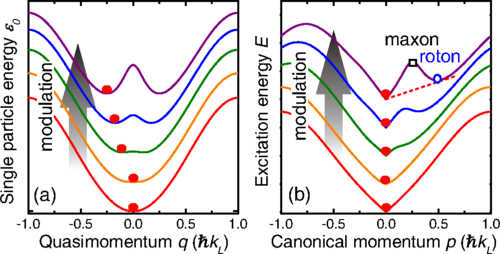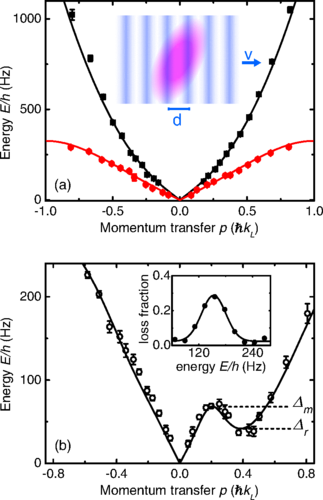# 2014 Cs Experiment

Roton-Maxon Excitation Spectrum of Bose Condensates in a Shaken Optical LatticeGeneration of roton-maxon dispersion in a shaken lattice. (a) For a single atom, the lattice modulation creates a double-well structure above a critical modulation amplitude (top three lines) . In our experiment, the atoms are prepared at the minimum with zero or negative momentum (q ≤ 0, red dot); see text. (b) With atomic interactions, a roton minimum (circle) and a maxon maximum (square) in the excitation spectrum can form. The dashed line indicates the critical velocity limited by the roton minimum according to the Landau criterion for superfluidity. Dispersions are upward offset with increasing modulation amplitude for clarity. The lattice reciprocal momentum is ℏkL=h/λ where λ is the wavelength of the lattice beams and h = 2πℏ is the Planck constant.Roton-Maxon excitation spectra. (a) We measure the excitation spectra with N0 = 30 000 atoms in a harmonic trap (square) and in a stationary lattice (circle) with DMD-based Bragg spectroscopy. The inset illustrates the moving optical potential with velocity v and periodicity d created by the DMD on the BEC (tilted ellipse); see text. The solid lines correspond to the Bogoliubov model with chemical potentials equal to the trapaveraged values. (b) For a BEC with N0 = 9000 atoms loaded in a shaken optical lattice, we measure the excitation spectrum along the lattice direction. The modulation amplitude (peak to peak) is Δx = 33 nm. The solid line is the best fit based on Eq. (1). The inset shows a typical atom loss spectrum taken at k = −0.38 kL. In both panels, the scattering length is a = 47 a0.